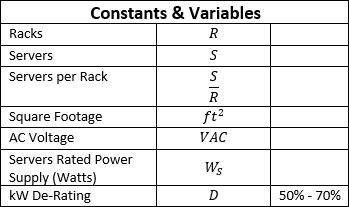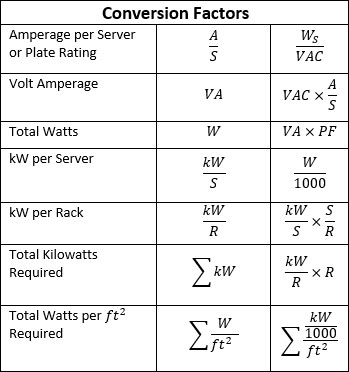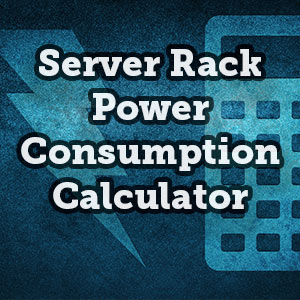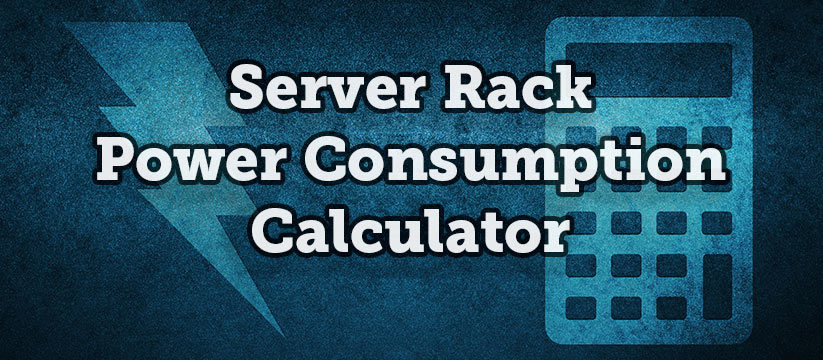# Server Rack Power Consumption Calculator

Commissioning a new data center and data center capacity planning are daunting tasks for even the most skilled data center manager. A lot of complex calculations need to be made to determine the configuration of the facility, if your current configuration is efficient, and if the facility is meeting the power consumption requirements of your data center. To help, we have created a handy server rack power consumption calculator tool to help you.

## How to Calculate Power Consumption of a Server Rack

Using the steps below, you can see how we made these calculations and understand where the results in the Server Rack Power Consumption Calculator are coming from.First, you need to know the useable Square Footage of your facility and the Power Supply for each Server (Server Watts). You will also need to find out your Facility Voltage (VAC), which will likely be 120V or 240V. Next, you need to determine the Number of Racks and the number of Servers Per Rack. If you don’t know any of these variables, you can easily find out by asking your data center staff.

### 2. Calculate Amps Per Server

The first and easiest calculation you need to make is Amps per Server, which will help you calculate the overall power draw per server. To do this, simply divide your Power Supply for Servers (Server Watts) by your Facility Power (VAC).

### 3. Calculate Maximum kW Per RackTo begin to calculate your Maximum kW Per Rack, you will first need to use the Number of Racks you already determined, or you can calculate Maximum Number of Racks you will be using. Do this by taking the usable floor space for the data center and dividing it by the amount of floor space each rack takes up, which will depend on your aisle arrangement. On a standard 8-pitch layout, that’s 16 ft2. We have added this to the bottom of the Server Rack Power Consumption Calculator to make it easy for you.

Next, determine the power supply of each server (Server Watts), which will be used to calculate the Kilowatts Per Server. This number is usually found written on the power supply of the server. Next,  divide the number of server watts by 1,000 to get kW Per Server.

After calculating the kW Per Server, you will then multiply that by the number of servers per rack, to get your final data center Maximum kW Per Rack.

### 4. Calculate Total Kilowatts

You’ve already done the legwork for this. To calculate Total Kilowatts needed, you want to multiply the number of servers per rack by kW Per Server. Use this number to calculate the Watts Per ft2.

### 5. Calculate Total Watts Per Square Foot

Finally, you need to calculate your Total Watts Per Square Foot. This is how much power your data center consumes per square foot. To get your Total Watts Per ft2 you will need to multiply the Total Kilowatts you calculated before and multiply it by 1,000. You will then divide that by the usable square footage of the facility (usually the amount of raised floor space). The result will be your Total Watts Per ft2.

### Rack Production Load: De-Rating and Plate Rating (Optional)

In addition to the basic calculations, we have included a way to get these calculations if the equipment is “De-Rated”. This is in case you would like to adjust the calculation based on the production load. For example, if your equipment is de-rated by 20%, your equipment is running at 80% production load. Equipment running at 100% is running at “Plate Rating”. When planning, you would consider your equipment to be running at Plate Rating as the worst-case scenario since it would be at full capacity. Whether you want to use this tool is entirely up to you. If you do not wish to use this feature, just leave it at 100.

## Use Case Scenario

For this example, let’s say you are on the board of directors for a big Real Estate Investment Trust (REIT) and you are in the process of buying a data center facility. You found one that looks like it might just be what you’re looking for and it’s in a decent location. It is now your job to see if this facility is a good deal. More importantly, you need to find out that it is capable of meeting your organization’s needs. You can use the server rack power consumption calculator above to follow along.

### Facility Specifications

The facility’s listing shows that it includes 32,874 ft2 with 13,903 ft2 of usable raised floor space. The listing shows the Facility Power to be 220 VAC and the building’s power capacity at 1.5 MW. In this scenario we will say that you are using 42U Server Cabinets with 15 Servers Per Rack with each server having a 750 W Power Supply, which we will call “Server Watts”.

### Making the Calculations

The first thing you need to do is calculate your Watts Per Square Foot (ft2). To do this, you will look at the facility’s power capacity which, as stated earlier, is 1.5 MW (1,500,000 W). Next, you will then divide 1,500,000 W by your usable square footage of 13,903 ft2 which equals 108 Watts per ft2.

Next, you need to calculate the Number of Racks that can fit in this space. On an 8-pitch layout, one rack requires 16 ft2. So, you would divide 13,903 ft2 by 16 ft2 which equals about 869 racks, which equals the maximum number of racks you can fit into the facility. Enter 869 racks into the calculator then leave the “De-Rating and/or Production Load” at 100% on the slider. 100% is considered to be at “Plate Rating”, or the maximum load that it can generate.

### The Verdict

After entering in all your variables, you can now see that we have finally calculated Amps per Server, kW per Rack, Total kW, and Total Watts Per ft2. Immediately, we see a problem: At Plate Rating, the Total Watts per ft2 is about 668 Watts when the facility is only capable of running at 108 Watts per ft2. To get to 108 Watts per ft2 you will either need to significantly de-rate the equipment or reduce the number of racks. In the event you reduce the number of racks, you have a large amount of unused floor space. However, if the equipment is de-rated, you will not be getting the most out of your equipment. In fact, in this scenario, you would have to drop the production load to about 20%. Your equipment is not getting much use at all in this scenario.

Based on these findings using the server rack power consumption calculator you can see that purchasing this facility will probably be a bad deal. Furthermore, in order for this facility to support current production loads, we would need to completely retrofit all of its electrical systems. Therefore, it would not only be incredibly expensive, but it’s also simply just not worth it.

SummaryArticle Name
Server Rack Power Consumption Calculator - RackSolutions
Description
Building out a new data center is a daunting task. To help you out, we've created a handy server rack power consumption calculator tool.
Author
Publisher Name
RackSolutions
Publisher Logo